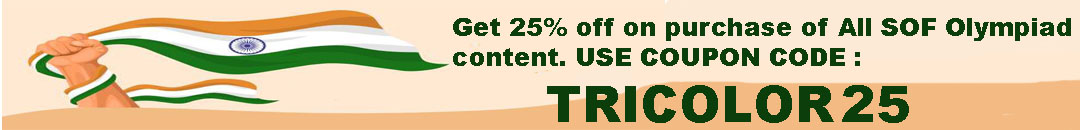# User ForumSubject :IMO    Class : Class 7

Class : Class 1

Subject :IMO    Class : Class 7

Class : Class 1

Subject :IMO    Class : Class 5

## Ans 1:

Class : Class 5
280 litre because 40 * 4 = 160 so 70 *4 is 280 litre

## Ans 2:

Class : Class 9
first divide 160 by 40=4 so 70 multiply by 40 = 280

Subject :IMO    Class : Class 5

## Ans 1:

Class : Class 9
The ans is b.1197

Subject :IMO    Class : Class 6

## Ans 1:

Class : Class 9
Plus sign means is smaller than so a is smaller than b ; b is smaller than c. That means c is smaller than a. = means is smaller than so c = a. answer is D

## Ans 2:

Class : Class 7

Subject :IMO    Class : Class 5

## Ans 1:

Class : Class 9
The ans is C

Subject :IMO    Class : Class 6

## Ans 1:

Class : Class 6
The formula is (L.C.M.*H .C.F.)/1st number.

Subject :IMO    Class : Class 4

## Ans 1:

Class : Class 4
ans a-80. From 1-9 there are 9 digits . so we are left with 151-9=142 digits. Note that from 10 onwards all numbers are of 2 digits. So there are 142/2=71 numbers to have 142 digits. Therefore there are 9+71=80 numbers.

Subject :IMO    Class : Class 4

## Ans 1:

Class : Class 5
The no is 31.31x5=155 209=364.Hence neither statement 1 nor statement 2 is correct

Class : Class 6

Class : Class 4

## Ans 4:

Class : Class 6
(364-209) divided by 5=3131*3=93So, the answer is C.

Class : Class 4

## Ans 6:

Class : Class 6
Answer should only and only be D

Class : Class 4
ans-d

## Ans 8:

Class : Class 4
Youâre are supposed to multiply the number by 3 hence the answer is C

Subject :IMO    Class : Class 5

Class : Class 7

Class : Class 5
C

Class : Class 9
The ans is C 48